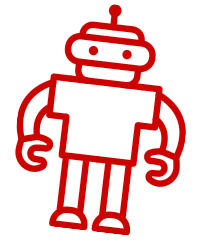## High School - Algebra 1

When students think of algebra, it’s usually the math that has both letters and numbers. Many would think it’s unnecessary and a headache, especially that there’s not just one but three algebra-related courses in high school. But come to think of it, algebra is actually very useful in real life.

One of the most useful things that algebra teaches is how to solve equations. For example, consider this: What number, when added to 3, makes 7? In algebraic notation, that would be x + 3 = 7. Solving that equation would give 4 as the value of x.

Algebra aims to simplify problems like that with a notation that can be operated on mathematically. It still uses the basic arithmetic operations of addition, subtraction, multiplication, and division to isolate the unknown quantity, which is usually denoted as x. Other letters can also be used if there is more than one unknown: y, z, a, b, c, and so on.

Another common theme in algebra is recognizing patterns. For example, the equations 4x + 2 = 10 and 3x + 7 = 15 may not be the same, but they do follow a similar form, which can be expressed as ax + b = c. The letters a, b, and c represent the numbers in each equation above.

Knowing the general form of different equations also makes it easier to solve for x. Once students know how to solve for x given form of equation, it can serve as a shortcut in solving other equations of the same form.

Solving word problems are also a lot easier when done with algebra. Any unknown quantity can be represented by letters, and if one unknown can be expressed in terms of the other, substitution can be done. This reduces the number of unknowns, thus simplifying the equation. With this and other similar techniques, students can level up their problem-solving skills.